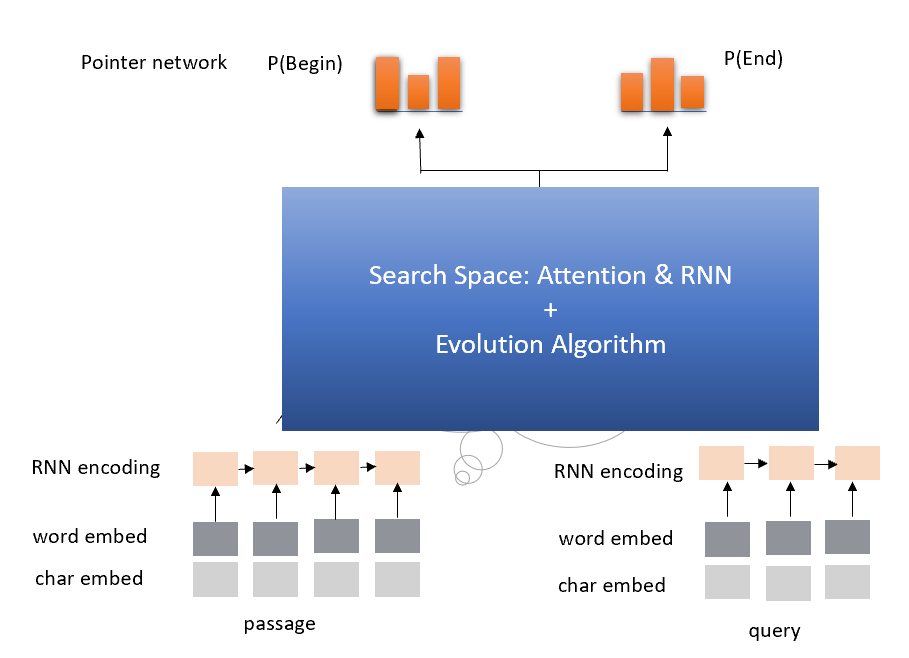Automatic Model Architecture Search for Reading Comprehension¶

This example shows us how to use Genetic Algorithm to find good model architectures for Reading Comprehension.

1. Search Space¶

Since attention and RNN have been proven effective in Reading Comprehension, we conclude the search space as follow:

1. IDENTITY (Effectively means keep training).

2. INSERT-RNN-LAYER (Inserts a LSTM. Comparing the performance of GRU and LSTM in our experiment, we decided to use LSTM here.)

3. REMOVE-RNN-LAYER

4. INSERT-ATTENTION-LAYER(Inserts an attention layer.)

5. REMOVE-ATTENTION-LAYER

6. ADD-SKIP (Identity between random layers).

7. REMOVE-SKIP (Removes random skip).New version¶

Also we have another version which time cost is less and performance is better. We will release soon.

2. How to run this example in local?¶

wget http://nlp.stanford.edu/data/glove.840B.300d.zip
unzip glove.840B.300d.zip

2.2 Update configuration¶

Modify nni/examples/trials/ga_squad/config.yml, here is the default configuration:

trialCommand: python3 trial.py

trialGpuNumber: 0
trialConcurrency: 1
maxTrialNumber: 10
maxExperimentDuration: 1h

searchSpace: {}  # hard-coded in tuner
tuner:
className: customer_tuner.CustomerTuner
codeDirectory: ~/nni/examples/tuners/ga_customer_tuner
classArgs:
optimize_mode: maximize

trainingService:
platform: local

In the trial part, if you want to use GPU to perform the architecture search, change trialGpuNum from 0 to 1. You need to increase the maxTrialNumber and maxExperimentDuration, according to how long you want to wait for the search result.

3. Technical details about the trial¶

3.1 How does it works¶

The evolution-algorithm based architecture for question answering has two different parts just like any other examples: the trial and the tuner.

3.2 The trial¶

The trial has a lot of different files, functions and classes. Here we will only give most of those files a brief introduction:

• attention.py contains an implementation for attention mechanism in Tensorflow.

• data.py contains functions for data preprocessing.

• evaluate.py contains the evaluation script.

• graph.py contains the definition of the computation graph.

• rnn.py contains an implementation for GRU in Tensorflow.

• train_model.py is a wrapper for the whole question answering model.

Among those files, trial.py and graph_to_tf.py are special.

graph_to_tf.py has a function named as graph_to_network, here is its skeleton code:

def graph_to_network(input1,
input2,
input1_lengths,
input2_lengths,
graph,
dropout_rate,
is_training,
rnn_units=256):
topology = graph.is_topology()
layers = dict()
layers_sequence_lengths = dict()
num_units = input1.get_shape().as_list()[-1]
layers = input1*tf.sqrt(tf.cast(num_units, tf.float32)) + \
layers = input2*tf.sqrt(tf.cast(num_units, tf.float32))
layers = dropout(layers, dropout_rate, is_training)
layers = dropout(layers, dropout_rate, is_training)
layers_sequence_lengths = input1_lengths
layers_sequence_lengths = input2_lengths
for _, topo_i in enumerate(topology):
if topo_i == '|':
continue
if graph.layers[topo_i].graph_type == LayerType.input.value:
# ......
elif graph.layers[topo_i].graph_type == LayerType.attention.value:
# ......
# More layers to handle

As we can see, this function is actually a compiler, that converts the internal model DAG configuration (which will be introduced in the Model configuration format section) graph, to a Tensorflow computation graph.

topology = graph.is_topology()

performs topological sorting on the internal graph representation, and the code inside the loop:

for _, topo_i in enumerate(topology):

performs actually conversion that maps each layer to a part in Tensorflow computation graph.

3.3 The tuner¶

The tuner is much more simple than the trial. They actually share the same graph.py. Besides, the tuner has a customer_tuner.py, the most important class in which is CustomerTuner:

class CustomerTuner(Tuner):
# ......

def generate_parameters(self, parameter_id):
"""Returns a set of trial graph config, as a serializable object.
parameter_id : int
"""
if len(self.population) <= 0:
logger.debug("the len of poplution lower than zero.")
raise Exception('The population is empty')
pos = -1
for i in range(len(self.population)):
if self.population[i].result == None:
pos = i
break
if pos != -1:
indiv = copy.deepcopy(self.population[pos])
self.population.pop(pos)
else:
random.shuffle(self.population)
if self.population.result > self.population.result:
self.population = self.population
indiv = copy.deepcopy(self.population)
self.population.pop(1)
indiv.mutation()
graph = indiv.config

# ......

As we can see, the overloaded method generate_parameters implements a pretty naive mutation algorithm. The code lines:

if self.population.result > self.population.result:
self.population = self.population
indiv = copy.deepcopy(self.population)

controls the mutation process. It will always take two random individuals in the population, only keeping and mutating the one with better result.

3.4 Model configuration format¶

Here is an example of the model configuration, which is passed from the tuner to the trial in the architecture search procedure.

{
"max_layer_num": 50,
"layers": [
{
"input_size": 0,
"type": 3,
"output_size": 1,
"input": [],
"size": "x",
"output": [4, 5],
"is_delete": false
},
{
"input_size": 0,
"type": 3,
"output_size": 1,
"input": [],
"size": "y",
"output": [4, 5],
"is_delete": false
},
{
"input_size": 1,
"type": 4,
"output_size": 0,
"input": ,
"size": "x",
"output": [],
"is_delete": false
},
{
"input_size": 1,
"type": 4,
"output_size": 0,
"input": ,
"size": "y",
"output": [],
"is_delete": false
},
{"Comment": "More layers will be here for actual graphs."}
]
}

Every model configuration will have a “layers” section, which is a JSON list of layer definitions. The definition of each layer is also a JSON object, where:

• type is the type of the layer. 0, 1, 2, 3, 4 corresponds to attention, self-attention, RNN, input and output layer respectively.

• size is the length of the output. “x”, “y” correspond to document length / question length, respectively.

• input_size is the number of inputs the layer has.

• input is the indices of layers taken as input of this layer.

• output is the indices of layers use this layer’s output as their input.

• is_delete means whether the layer is still available.